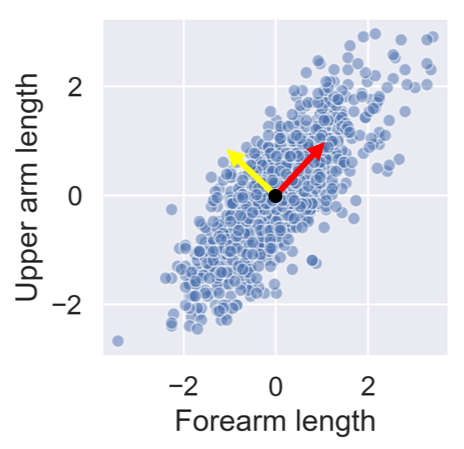# Principal component intuitionAfter standardizing the lower and upper arm lengths from the ANSUR dataset we've added two perpendicular vectors that are aligned with the main directions of variance. We can describe each point in the dataset as a combination of these two vectors multiplied with a value each. These values are then called principal components.

Which of the following statements is true?